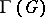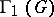# Graph automorphism

(diff) ← Older revision | Latest revision (diff) | Newer revision → (diff)
An isomorphic mapping of a graph onto itself (cf. Graph isomorphism). The set of all automorphisms of a given graph forms a group with respect to the operation of composition of automorphisms. The automorphisms of a graphgenerate a groupof permutations of vertices, which is called the group (or vertex group) of, and a group of edge permutations, called the edge group of. The edge group and vertex group of a graphwithout loops and multiple edges are isomorphic if and only ifcontains not more than one isolated vertex and if none of its connected components is an isolated edge. For each finite groupthere exists a graph whose automorphism group is isomorphic to. There also exist permutation groups on a set ofelements which are not the vertex group of any graph withvertices. Various types and measures of symmetry of a graph can be related to its automorphisms. A graph with no automorphisms other than the identical one is said to be asymmetric. If, almost all graphs withvertices are asymmetric.